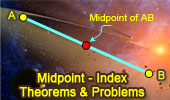# Midpoint, Theorems and Problems - Table of Content (Page 9)Proposed Problem 123. Area of triangle, Similarity, Trisection of Sides. Midpoints.

Proposed Problem 122. Marion Walter's Theorem. Area of triangle and Hexagon, Trisection of Sides. Midpoints.

Proposed Problem 121. Similarity and Area of triangle, Trisection of Sides.
Midpoints.

Proposed Problem 108. Angles, Triangle, Median. Midpoints.

Proposed Problem 98. Quadrilateral Area, Centroid, Similarity.
Midpoints.

Proposed Problem 90. Quadrilateral and Triangle Areas, Midpoints.

Proposed Problem 89. Triangles of equal area, Midpoints.

Proposed Problem 88. Triangle and Quadrilateral Areas, Midpoints.

Proposed Problem 87. Area of Quadrilaterals and Midpoints of Diagonals.

Proposed Problem 1: Angles of a Triangle. Midpoint, Congruence. Hints are provided.

Proposed Problem 78: Angles of a Circle. Perpendicular and parallel lines, Midpoint, Diameter, Chord, Cyclic quadrilateral, Congruence.

Proposed Problem 61: Triangle, Trisection of Sides. Midpoints.

Go to Page: Previous | 1 | 2 | 3 | 4 | 5 | 6 | 7 | 8 | 9 | 10 | Next

 Home | Search | Geometry | Post a comment | Email | by Antonio Gutierrez